# Chapter 4: Analyzing Geomodel Topology¶

import gempy as gp
from gempy.assets import topology as tp

import numpy as np
import matplotlib.pyplot as plt

import warnings
warnings.filterwarnings("ignore")


First let’s set up a very simple example model. For that we initialize the geo_data object with the correct model extent and the resolution we like. Then we load our data points from csv files and set the series and order the formations (stratigraphic pile).

geo_model = gp.create_model("Model_Tutorial6")

data_path = 'https://raw.githubusercontent.com/cgre-aachen/gempy_data/master/'
gp.init_data(
geo_model, [0, 3000, 0, 20, 0, 2000], [50, 10, 67],
path_i=data_path+"data/input_data/tut_chapter6/ch6_data_interf.csv",
path_o=data_path+"data/input_data/tut_chapter6/ch6_data_fol.csv"
)
gp.map_stack_to_surfaces(
geo_model,
{
"fault": "Fault",
"Rest": ('Layer 2', 'Layer 3', 'Layer 4', 'Layer 5')
}
)
geo_model.set_is_fault(["fault"]);
gp.set_interpolator(geo_model)
sol = gp.compute_model(geo_model, compute_mesh=True)


Out:

Active grids: ['regular']
Fault colors changed. If you do not like this behavior, set change_color to False.
Setting kriging parameters to their default values.
Compiling theano function...
Level of Optimization:  fast_compile
Device:  cpu
Precision:  float64
Number of faults:  1
Compilation Done!
Kriging values:
values
range              3605.61
$C_o$            309533.33
drift equations  [3, 3, 3]

gp.plot_2d(geo_model, cell_number=)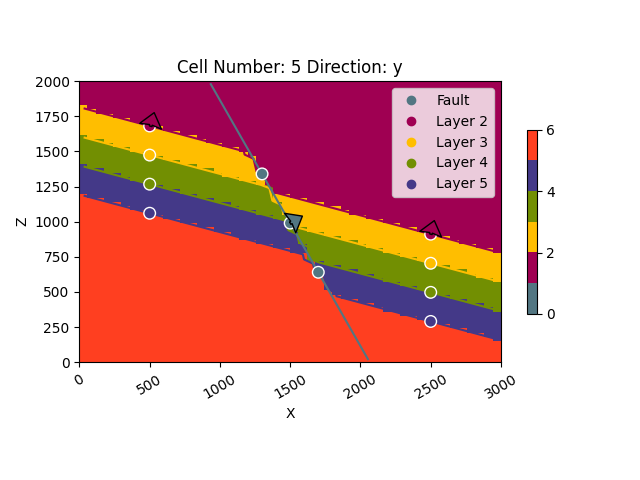Out:

<gempy.plot.visualization_2d.Plot2D object at 0x7fcb84e4ff70>


## Analyzing Topology¶

GemPy sports in-built functionality to analyze the topology of its models. All we need for this is our geo_data object, lithology block and the fault block. We input those into gp.topology_compute and get several useful outputs:

• an adjacency graph G, representing the topological relationships of the model

• the centroids of the all the unique topological regions in the model (x,y,z coordinates of their center)

• a list of all the unique labels (labels_unique)

• two look-up-tables from the lithology id’s to the node labels, and vice versa

edges, centroids = tp.compute_topology(geo_model)


The first output of the topology function is the set of edges representing topology relationships between unique geobodies of the block model. An edge is represented by a tuple of two int geobody (or node) labels:

edges


Out:

{(9, 10), (4, 10), (1, 2), (3, 4), (1, 8), (3, 10), (2, 3), (2, 9), (1, 7), (4, 5), (3, 9), (5, 10), (6, 7), (8, 9), (1, 6), (7, 8), (2, 8)}


The second output is the centroids dict, mapping the unique geobody id’s (graph node id’s) to the geobody centroid position in grid coordinates:

centroids


Out:

{1: array([35.27893175,  4.5       , 50.19485658]), 2: array([36.46666667,  4.5       , 29.14444444]), 3: array([37.59756098,  4.5       , 21.62195122]), 4: array([38.84563758,  4.5       , 14.00671141]), 5: array([39.09550562,  4.5       ,  5.37640449]), 6: array([ 9.79081633,  4.5       , 60.10204082]), 7: array([10.17687075,  4.5       , 51.02721088]), 8: array([11.37804878,  4.5       , 43.47560976]), 9: array([12.51098901,  4.5       , 35.90659341]), 10: array([13.659857  ,  4.5       , 15.34320735])}


After computing the model topology, we can overlay the topology graph over a model section:

# 2-D Visualization of the Topology Graph¶

gp.plot.plot_topology(geo_model, edges, centroids)
plt.show()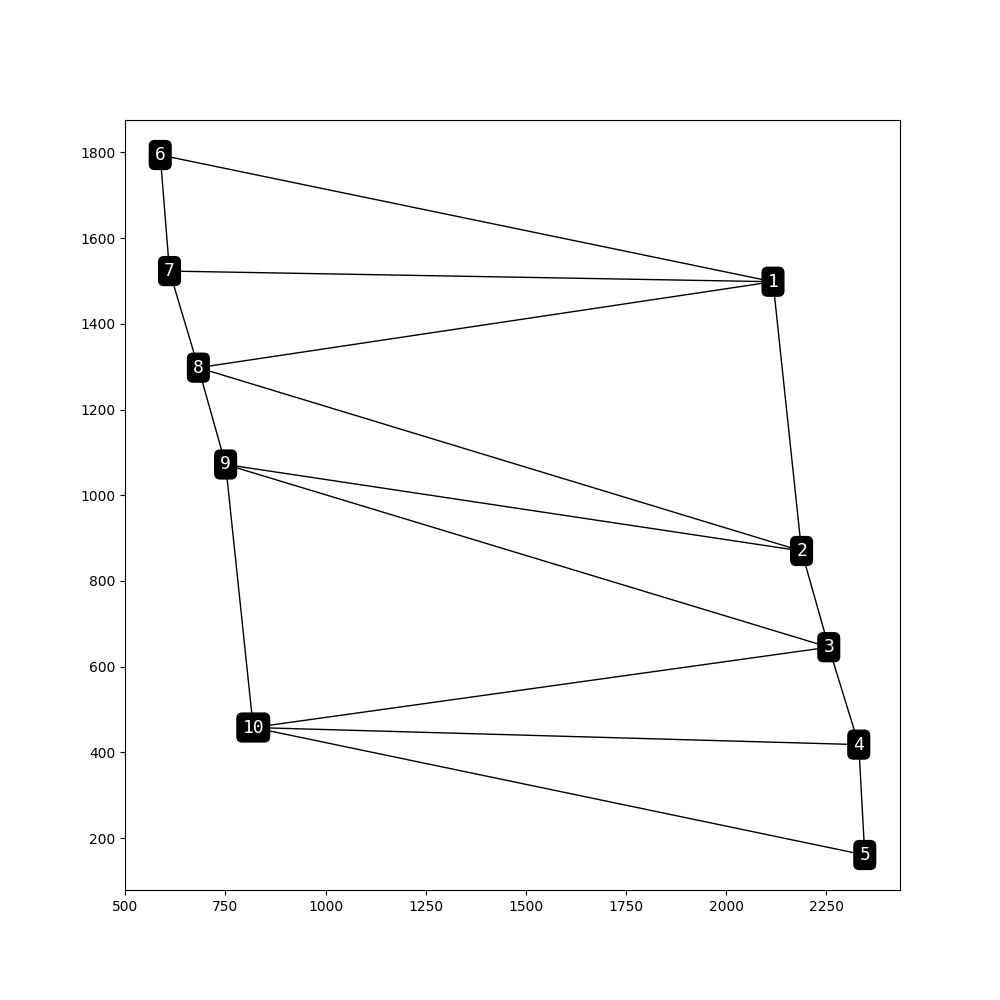gp.plot_2d(geo_model, cell_number=, show=False)
gp.plot.plot_topology(geo_model, edges, centroids, scale=True)
plt.show()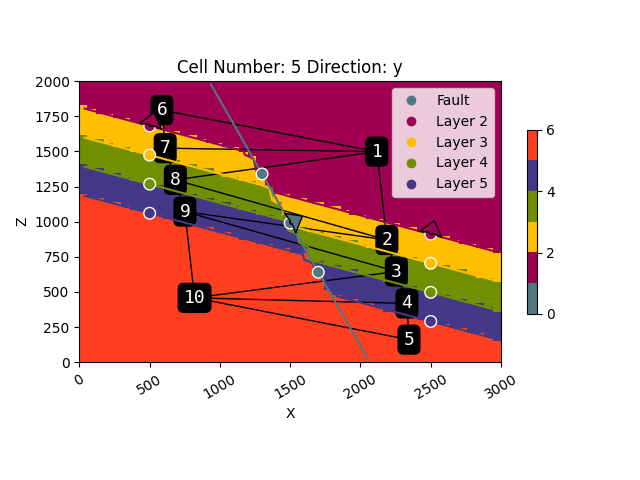Another way to encode and visualize the geomodel topology is using an adjacency graph:

M = tp.get_adjacency_matrix(geo_model, edges, centroids)
print(M)


Out:

[[False  True False False False  True  True  True False False]
[ True False  True False False False False  True  True False]
[False  True False  True False False False False  True  True]
[False False  True False  True False False False False  True]
[False False False  True False False False False False  True]
[ True False False False False False  True False False False]
[ True False False False False  True False  True False False]
[ True  True False False False False  True False  True False]
[False  True  True False False False False  True False  True]
[False False  True  True  True False False False  True False]]

tp.plot_adjacency_matrix(geo_model, M)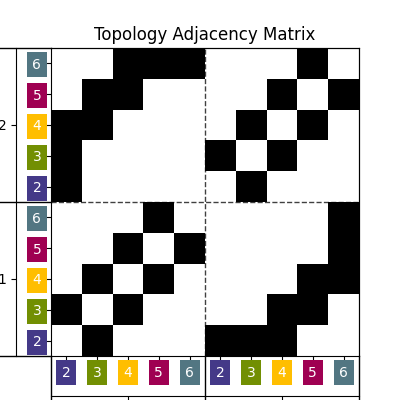# 3-D Visualization of the Topology Graph¶

You can also plot the topology in 3-D using GemPy’s 3-D visualization toolkit powered by pyvista:

from gempy.plot._vista import Vista
gpv = Vista(geo_model)
gpv.plot_topology(edges, centroids)
gpv.show()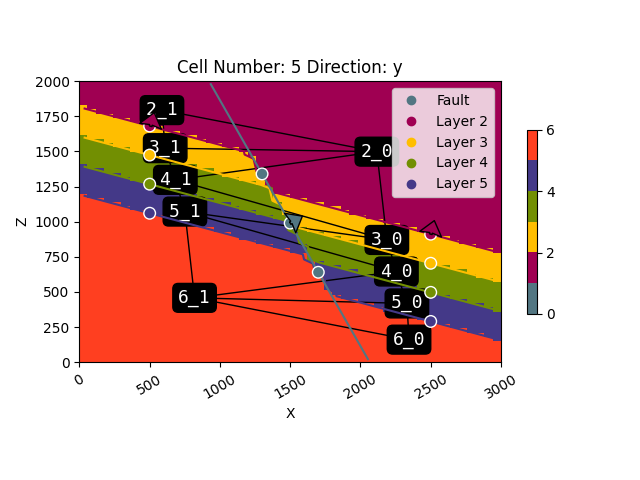Out:

surface
Fault       #527682
Layer 2     #9f0052
Layer 3     #ffbe00
Layer 4     #728f02
Layer 5     #443988
basement    #ff3f20
Name: color, dtype: object


# Look-up tables¶

The topology asset provides several look-up tables to work with the unique geobody topology id’s.

Mapping node id’s back to lithology / surface id’s:

lith_lot = tp.get_lot_node_to_lith_id(geo_model, centroids)
lith_lot


Out:

{1: 2, 2: 3, 3: 4, 4: 5, 5: 6, 6: 2, 7: 3, 8: 4, 9: 5, 10: 6}


Figuring out which nodes are in which fault block:

fault_lot = tp.get_lot_node_to_fault_block(geo_model, centroids)
fault_lot


Out:

{1: 0, 2: 0, 3: 0, 4: 0, 5: 0, 6: 1, 7: 1, 8: 1, 9: 1, 10: 1}


We can also easily map the lithology id to the corresponding topology id’s:

tp.get_lot_lith_to_node_id(lith_lot)


Out:

{2: [1, 6], 3: [2, 7], 4: [3, 8], 5: [4, 9], 6: [5, 10]}


# Detailed node labeling¶

sphinx_gallery_thumbnail_number = 4

dedges, dcentroids = tp.get_detailed_labels(geo_model, edges, centroids)
gp.plot_2d(geo_model, cell_number=, show=False)
gp.plot.plot_topology(geo_model, dedges, dcentroids, scale=True)
plt.show()dedges


Out:

{('3_0', '4_0'), ('3_1', '4_1'), ('2_0', '2_1'), ('4_1', '5_1'), ('6_0', '6_1'), ('4_0', '6_1'), ('4_0', '5_1'), ('2_0', '4_1'), ('2_0', '3_1'), ('2_0', '3_0'), ('5_0', '6_1'), ('3_0', '5_1'), ('5_0', '6_0'), ('2_1', '3_1'), ('3_0', '4_1'), ('5_1', '6_1'), ('4_0', '5_0')}

dcentroids


Out:

{'2_0': array([35.27893175,  4.5       , 50.19485658]), '3_0': array([36.46666667,  4.5       , 29.14444444]), '4_0': array([37.59756098,  4.5       , 21.62195122]), '5_0': array([38.84563758,  4.5       , 14.00671141]), '6_0': array([39.09550562,  4.5       ,  5.37640449]), '2_1': array([ 9.79081633,  4.5       , 60.10204082]), '3_1': array([10.17687075,  4.5       , 51.02721088]), '4_1': array([11.37804878,  4.5       , 43.47560976]), '5_1': array([12.51098901,  4.5       , 35.90659341]), '6_1': array([13.659857  ,  4.5       , 15.34320735])}


So lets say we want to check if the purple layer (id 5) is connected across the fault to the yellow layer (id 3). For this we can make easy use of the detailed labeling and the check_adjacency function:

tp.check_adjacency(dedges, "5_1", "3_0")


Out:

True


We can also check all geobodies that are adjacent to the purple layer (id 5) on the left side of the fault (fault id 1):

tp.get_adjacencies(dedges, "5_1")


Out:

{'6_1', '4_1', '4_0', '3_0'}


Total running time of the script: ( 0 minutes 5.379 seconds)

Gallery generated by Sphinx-Gallery